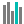#BETA.INV (PQL - xl)

This function calculates the inverse of the cumulative beta distribution function

• Library: PQL \ Spreadsheet \ Statistical
• Compatibility: Any content (regardless of data source) in the Tabulate spreadsheet module
• Solve: This function can be used with the Solve plug-in: both as part of the objective function and constraint functions

#### Syntax

BETA.INV(probability, alpha, beta, OPTIONAL A, OPTIONAL B)

##### Function Arguments
 Name Description Type Optional probability Probability of the distribution Number alpha Alpha parameter of the distribution Number beta Beta parameter of the distribution Number A Lower bound of the interval for x; 0 if omitted Number Y B Upper bound of the interval for x; 1 if omitted Number Y